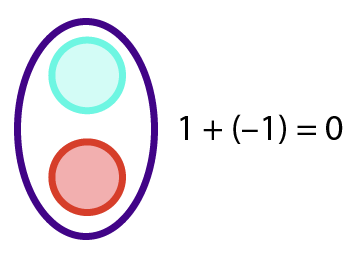## Notation and Modeling Addition of Integers

### Learning Outcomes

• Model addition of integers with color counters

Now that we have located positive and negative numbers on the number line, it is time to discuss arithmetic operations with integers.

Most students are comfortable with the addition and subtraction facts for positive numbers. But doing addition or subtraction with both positive and negative numbers may be more difficult. This difficulty relates to the way the brain learns.

The brain learns best by working with objects in the real world and then generalizing to abstract concepts. Toddlers learn quickly that if they have two cookies and their older brother steals one, they have only one left. This is a concrete example of $2 - 1$. Children learn their basic addition and subtraction facts from experiences in their everyday lives. Eventually, they know the number facts without relying on cookies.

Addition and subtraction of negative numbers have fewer real world examples that are meaningful to us. Math teachers have several different approaches, such as number lines, banking, temperatures, and so on, to make these concepts real.

We will model addition and subtraction of negatives with two color counters. We let a blue counter represent a positive and a red counter will represent a negative.If we have one positive and one negative counter, the value of the pair is zero. They form a neutral pair. The value of this neutral pair is zero as summarized in the figure below.

A blue counter represents $+1$. A red counter represents $-1$. Together they add to zero.In the following examples, we will model four different addition facts using the numbers $5,-5\text{ and }3,-3$.

### example

Model: $5+3$

Solution:

 Interpret the expression. $5+3$ means the sum of $5$ and $3$ . Model the first number. Start with $5$ positives.Model the second number. Add $3$ positives.Count the total number of counters.The sum of $5$ and $3$ is $8$. $5+3=8$

### try it

Model the expression.

$2+4$

Model the expression.

$2+5$

### example

Model: $-5+\left(-3\right)$

### try it

Model the expression.

$-2+\left(-4\right)$

Model the expression.

$-2+\left(-5\right)$

The first and second examples are very similar. The first example adds $5$ positives and $3$ positives—both positives. The second example adds $5$ negatives and $3$ negatives—both negatives. In each case, we got a result of $8$, either $8$ positives or $8$ negatives. When the signs are the same, the counters are all the same color.

Now let’s see what happens when the signs are different.

### example

Model: $-5+3$

Notice that there were more negatives than positives, so the result is negative.

### try it

Model the expression, and then simplify:
$2+\left(-4\right)$

Model the expression, and then simplify:
$2+\left(-5\right)$

### example

Model: $5+\left(-3\right)$

### try it

Model the expression, and then simplify:

$\left(-2\right)+4$

Model the expression:
$\left(-2\right)+5$

### example

1. $4 + 2$
2. $−3 + 6$
3. $4 + (−5)$
4. $-2 + (−3)$

### try it

1. $3 + 4$
2. $−1 + 4$
3. $4 + (−6)$
4. $−2 + (−2)$
1. $5 + 1$
2. $−3 + 7$
3. $2 + (−8)$
4. $−3 + (−4)$